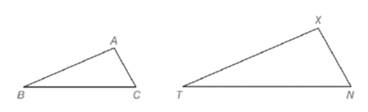Chapter 5.2, Problem 35E### Elementary Geometry for College St...

6th Edition
Daniel C. Alexander + 1 other
ISBN: 9781285195698

#### Solutions

Chapter
Section### Elementary Geometry for College St...

6th Edition
Daniel C. Alexander + 1 other
ISBN: 9781285195698
Textbook Problem
143 views

# Given △ A B C , a second triangle ( △ X T N ) is constructed so that ∠ X ≅ ∠ A and ∠ N ≅ ∠ C . a) Is ∠ T congruent to ∠ B ? b) Using intuition (appearance), does it seem that △ X T N is similar to △ A B C ?To determine

a)

Whether T is congruent to B.

Explanation

Approach:

The sum of measure of three angle in a triangle is 180.

Given:

It is given that XA and NC.

Calculation:

In ΔABC,

mA+mB+mC=180mB=180mCmA     (1)

In ΔXTN,

To determine

b)

Whether it seems that ΔABC is similar to ΔXTN.

### Still sussing out bartleby?

Check out a sample textbook solution.

See a sample solution

#### The Solution to Your Study Problems

Bartleby provides explanations to thousands of textbook problems written by our experts, many with advanced degrees!

Get Started